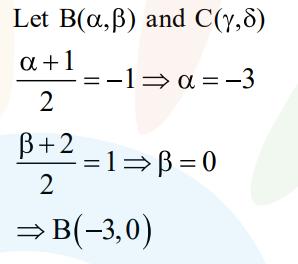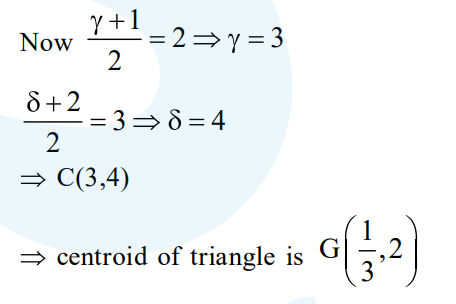# A triangle has a vertex at (1,2) and the mid pointsQuestion:

A triangle has a vertex at $(1,2)$ and the mid points of the two sides through it are $(-1,1)$ and $(2,3)$. Then the centroid of this triangle is :

1. $\left(\frac{1}{3}, 1\right)$

2. $\left(\frac{1}{3}, 2\right)$

3. $\left(1, \frac{7}{3}\right)$

4. $\left(\frac{1}{3}, \frac{5}{3}\right)$

Correct Option: , 2

Solution: